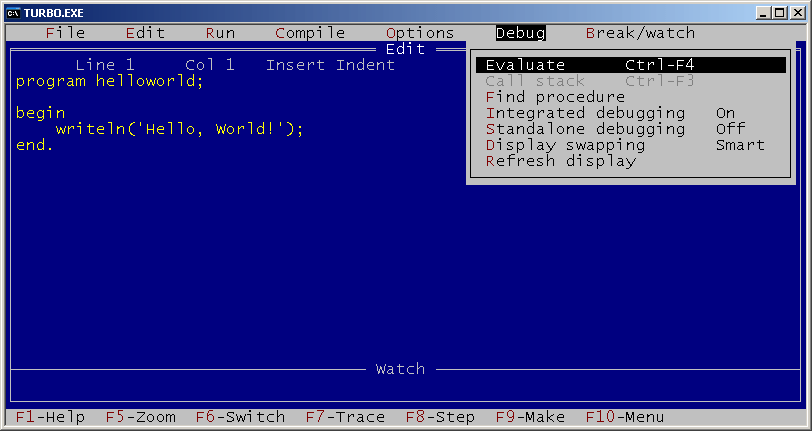# Turbo Pascal 5.5

Version of implementation Turbo Pascal of programming language Pascal

Turbo Pascal 5.5 was released on May 2, 1989.

Implemented language provided next major enhancement — basic support for object-oriented programming, including the concept of classes, static and dynamic objects, constructors and destructors and inheritance.

As for the interface, it finally introduced the distinctive blue full-screen user interface with pull-down menus, used in all later Turbo products. The IDE provided two more important features — step-by-step debugger and context-sensitive help with description of all built-in functions and possibility of copying fragments of code from help window and pasting it to your code.Turbo Pascal 5.5: general view

## Examples:

### Fibonacci numbers - Pascal (58):

This example uses recursive definition of Fibonacci numbers.

``````program fibonacci;

function fib(n:integer): integer;
begin
if (n <= 2) then
fib := 1
else
fib := fib(n-1) + fib(n-2);
end;

var
i:integer;

begin
for i := 1 to 16 do
write(fib(i), ', ');
writeln('...');
end.
``````

### Factorial - Pascal (44):

This example uses recursive factorial definition.

Note that this example works in all given implementations of Pascal, but it produces slightly different results. In gpc everything works perfectly. Turbo Pascal and Free Pascal have arithmetic overflow for factorial of numbers greater than 12, but Free Pascal reports an error:

13! = Runtime error 215 at \$004013C7
\$004013C7
\$00401449
\$004063E0

while Turbo Pascal doesn’t detect the error and simply prints wrong values:

13! = 1932053504
14! = 1278945280
15! = 2004310016
16! = 2004189184

This example doesn’t work in Turbo Pascal 3.0 and earlier due to absence of `longint` data type in these versions.

In GNU Pascal this program works without any problems.

``````program factorial;

function fact(n: integer): longint;
begin
if (n = 0) then
fact := 1
else
fact := n * fact(n - 1);
end;

var
n: integer;

begin
for n := 0 to 16 do
writeln(n, '! = ', fact(n));
end.
``````

### Hello, World! - Pascal (57):

``````program helloworld;

begin
writeln('Hello, World!');
end.
``````

### Factorial - Pascal (96):

This example is exactly the same as main recursive example for Pascal implementations, except for that it uses `real` data type to store factorial values. Command `writeln(f:-1:0)` outputs the floating point number `f` with 0 digits after decimal point and left-justifies it.

``````program factorial;

function fact(n: integer): real;
begin
if (n = 0) then
fact := 1
else
fact := n * fact(n - 1);
end;

var
n: integer;

begin
for n := 0 to 16 do
writeln(n, '! = ', fact(n):-1:0);
end.
``````

### CamelCase - Pascal (284):

This example processes the string char by char, and works with ASCII-codes to figure out whether they are lower- or uppercase letters. `ord` returns ASCII-code of a character, while `chr` converts given ASCII-code into a character. String capacity is omitted and thus set to 255 by default.

Note that in Turbo Pascal series this program works only with Turbo Pascal 4.0 and higher due to the fact that earlier versions didn’t have `char` datatype.

``````program Camelcase;

var
text, cc: string;
c: char;
i: integer;
lastSpace: boolean;

begin
lastSpace := true;
cc := '';
for i := 1 to Length(text) do
begin
c := text[i];
if ((c >= #65) and (c <= #90)) or ((c >= #97) and (c <= #122)) then
begin
if (lastSpace) then
begin
if ((c >= #97) and (c <= #122)) then
c := chr(ord(c) - 32);
end
else
if ((c >= #65) and (c <= #90)) then
c := chr(ord(c) + 32);
cc := cc + c;
lastSpace := false;
end
else
lastSpace := true;
end;
writeln(cc);
end.
``````

### CamelCase - Pascal (285):

This example is similar to previous one, but uses sets of characters for letter check. This makes the code more readable.

Note that in Turbo Pascal series this program works only with Turbo Pascal 4.0 and higher due to the fact that earlier versions didn’t have `char` datatype.

``````program Camelcase;

var
text, cc: string;
c: char;
i: integer;
lastSpace: boolean;
upper, lower: set of char;

begin
upper := ['A'..'Z'];
lower := ['a'..'z'];
lastSpace := true;
cc := '';
for i := 1 to Length(text) do
begin
c := text[i];
if (c in lower) or (c in upper) then
begin
if (lastSpace) then { convert to uppercase }
begin
if (c in lower) then
c := chr(ord(c) - 32);
end
else { convert to lowercase }
if (c in upper) then
c := chr(ord(c) + 32);
cc := cc + c;
lastSpace := false;
end
else
lastSpace := true;
end;
writeln(cc);
end.
``````